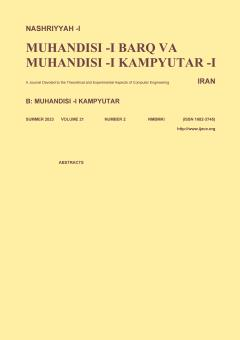• Home
• msedighi msedighi
• ### Current Issue### Browse

• #### List of Articlesmsedighi msedighi

• Open Access Article

1 - Quantum-Logic Synthesis Using Improved Block-Based Approach
K. Marjoei M. Houshmand M. Saheb Zamani M. Sedighi
Quantum-logic synthesis refers to generating a quantum circuit for a given arbitrary quantum gate according to a specific universal gate library implementable in quantum technologies. Previously, an approach called block-based quantum decomposition (BQD) has been propos More
Quantum-logic synthesis refers to generating a quantum circuit for a given arbitrary quantum gate according to a specific universal gate library implementable in quantum technologies. Previously, an approach called block-based quantum decomposition (BQD) has been proposed to synthesize quantum circuits by using a combination of two well-known quantum circuit synthesis methods, namely, quantum Shannon decomposition (QSD) and cosine-sine decomposition (CSD). In this paper, an improved block-based quantum decomposition (IBQD) is proposed. IBQD is a parametric approach and explores a larger space than CSD, QSD, and BQD to obtain best results for various synthesis cost metrics. IBQD cost functions for synthesis are calculated in terms of different synthesis cost metrics with respect to the parameters of the proposed approach. Furthermore, in order to find optimum results according to these functions, IBQD synthesis approach is defined as a constrained-optimization model. The results show that IBQD can lead to the minimum total gate cost among all the proposed approaches for the specific case of 4-qubit quantum circuit synthesis. Moreover, for the first time, the depth costs of the CSD, QSD, BQD, and IBQD synthesis approaches are evaluated and it is shown that IBQD makes a trade-off between the total gates and depth costs for the synthesized quantum circuits. Manuscript profile
• Open Access Article

2 - Optimizing Quantum Circuits by One-Way Quantum Computation Model Based on Pattern Geometries
M. Eslamy M. Saheb Zamani M. Sedighi M. Houshmand
A fundamentally quantum model of computation based on quantum entanglement and quantum measurement is called one-way quantum computation model (1WQC). Computations are shown by measurement patterns (or simply patterns) in this model where an initial highly entangled sta More
A fundamentally quantum model of computation based on quantum entanglement and quantum measurement is called one-way quantum computation model (1WQC). Computations are shown by measurement patterns (or simply patterns) in this model where an initial highly entangled state called a graph state is used to perform universal quantum computations. This graph together with the set of its input and output qubits is called the geometry of the pattern. Moreover, some optimization techniques have been introduced to simplify patterns. Previously, the 1WQC model has been applied to optimize quantum circuits. An approach for parallelizing quantum circuits has been proposed which takes a quantum circuit and then produces the corresponding pattern after performing the proposed optimization techniques for this model. Then it translates the optimized 1WQC patterns back to quantum circuits to parallelize the initial quantum circuit by using a set of rewriting rules. To improve previous works, in this paper, a new automatic approach is proposed to optimize patterns based on their geometries instead of using rewriting rules by applying optimization techniques simultaneously. Moreover, the optimized pattern is translated back to a quantum circuit and then this circuit is simplified by decreasing the number of auxiliary qubits. Results show that the quantum circuit cost metrics of the proposed approach is improved as compared to the previous ones. Manuscript profile
• Open Access Article

3 - The Effect of Granularity on the Design of Decimal Arithmetic Reconfigurable Units
S. Emami M. Sedighi
Recently, decimal arithmetic has received renewed attention in certain application domains such as financial computations. This is mostly due to the demand for more accurate decimal number representation and calculation in those applications. While decimal arithmetic ma More
Recently, decimal arithmetic has received renewed attention in certain application domains such as financial computations. This is mostly due to the demand for more accurate decimal number representation and calculation in those applications. While decimal arithmetic may be implemented in software and hardware, the latter form offers higher speeds and better performance. Traditionally, hardware decimal units have been designed as application-specific specialized hardware modules. However, emerging designs have come with some degree of reconfigurablility. But there is no research on the effects of reconfigurability parameters, such as granularity and degree of flexibility, on the overall characteristics of decimal hardware modules .In this paper, it will be shown that bit-level granularity is not suitable for decimal reconfigurable adders. Instead, digit-level granularity will lead to superior designs. The synthesis results indicate that increasing granularity level provides an area improvement of %12 and power improvement of %13.4. Unlike adders, increasing the granularity of decimal multipliers has an adverse effect on their quality and may cause up to %75 increase in their area and power consumption. Manuscript profile